## 基/坐标变换和图像压缩

### 基变换

• 对一个行向量组成的矩阵，左乘一个矩阵，就是对行向量进行线性组合；
• 对一个列向量组成的矩阵，右乘一个矩阵，就是对列向量进行线性组合；
• 对上面两种情形，各自转置，那么行向量的线性组合变为列向量的线性组合，列向量的线性组合变成了行向量的线性组合。$$P_{s \times s}=\left(\begin{array}{cccc} p_{11} & p_{12} & \cdots & p_{1 s} \\ p_{21} & p_{22} & \cdots & p_{2 s} \\ \vdots & \vdots & \ddots & \vdots \\ p_{s 1} & p_{s 2} & \cdots & p_{s s} \end{array}\right) \quad\left\{\begin{array}{l} \boldsymbol{n}_1=p_{11} \boldsymbol{m}_1+p_{21} \boldsymbol{m}_2+\cdots+p_{s 1} \boldsymbol{m}_{\boldsymbol{s}} \\ \boldsymbol{n}_2=p_{12} \boldsymbol{m}_1+p_{22} \boldsymbol{m}_2+\cdots+p_{s 2} \boldsymbol{m}_{\boldsymbol{s}} \\ \cdots \cdots \cdots \\ \boldsymbol{n}_{\boldsymbol{s}}=p_{1 s} \boldsymbol{m}_1+p_{2 s} \boldsymbol{m}_2+\cdots+p_{s s} \boldsymbol{m}_{\boldsymbol{s}} \end{array}\right.$$等价描述：

• 当且仅当向量$$\boldsymbol{n}_1, \boldsymbol{n}_2, \ldots, \boldsymbol{n}_s$$是基$$\boldsymbol{m}_1, \boldsymbol{m}_2, \ldots, \boldsymbol{m}_s$$的线性组合时，或者说向量$$\boldsymbol{n}_1, \boldsymbol{n}_2, \ldots, \boldsymbol{n}_s$$在基$$\boldsymbol{m}_1, \boldsymbol{m}_2, \ldots, \boldsymbol{m}_s$$张成的空间中时，上述方程才有解；
• 当这两个基是同一个向量空间的基时，才有过渡矩阵$$P$$。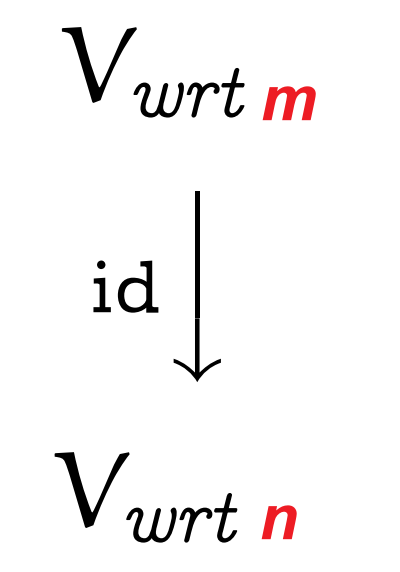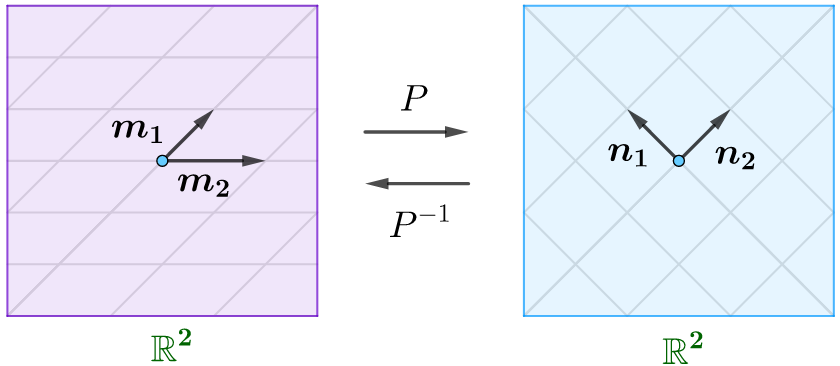### 坐标变换

• 可以快速求逆矩阵：
• 比如FFT矩阵为复数域上的酉矩阵(正交矩阵)，所以其共轭转置就是逆矩阵；
• 小波基，也是正交矩阵，所以转置一下就是其逆矩阵，JPEG2000用的就是这种；
• 少量的基向量就可以得到近似信号，可压缩的比例就比较高。

## 相似矩阵和若尔当标准型

### 相似矩阵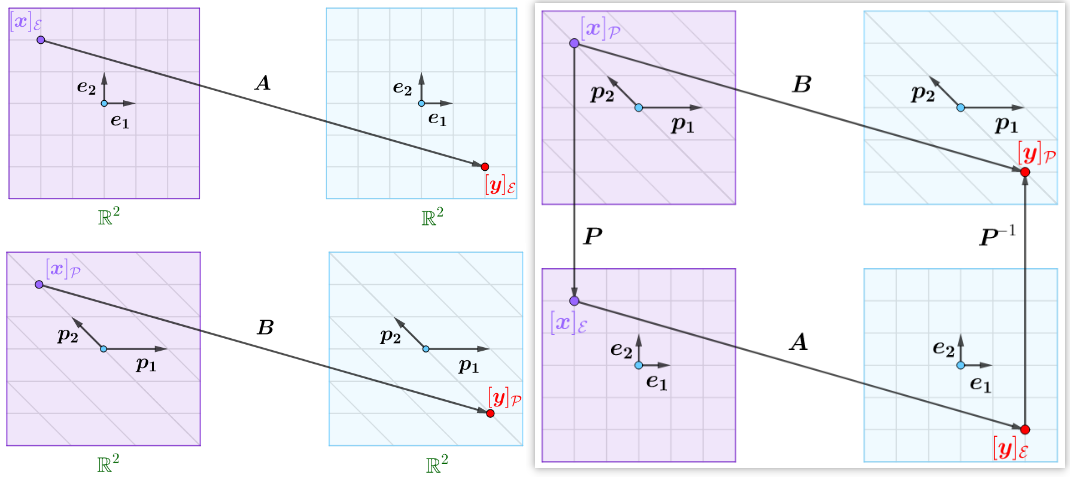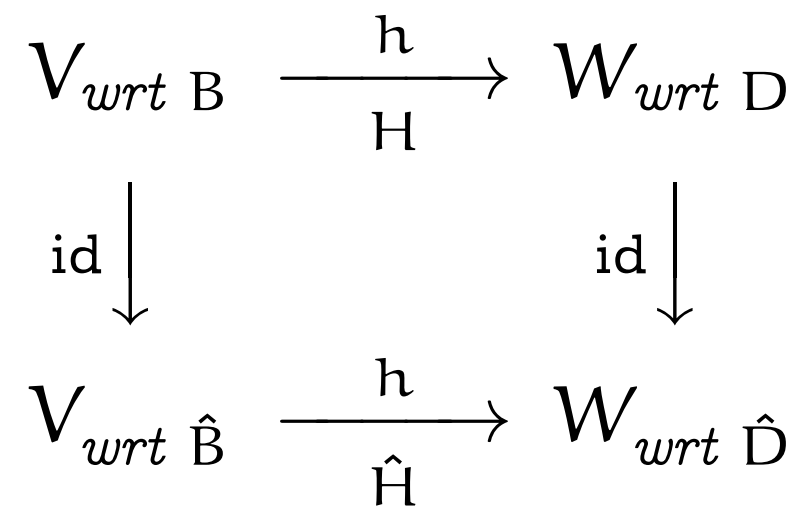(1) 相似矩阵—马同学
(2) 坐标变换—马同学
(3) 过渡矩阵—马同学

### 矩阵的分类—对角化

• 特征值都没有重根，矩阵很容易对角化并且找到对应的特征向量。
• 特征值有重根，如果一个矩阵的特征值$$\lambda_{1}=\lambda_{2}=4$$，那么可能存在下面两种情况：
• 可对角化，存在两个特征向量 (small family的情况)。$$\left[\begin{array}{ll} 4 & 0 \\ 0 & 4 \end{array}\right]$$只和自己相似，任意相似变换都得到自己$$M^{-1}\left[\begin{array}{ll} 4 & 0 \\ 0 & 4 \end{array}\right] M=4 M^{-1} I M=\left[\begin{array}{ll} 4 & 0 \\ 0 & 4 \end{array}\right]$$。
• 不能对角化，只有一个特征向量，也就是我们之前提到的退化矩阵 (big family 的情况，除上面情况之外的所有情形)。比如最典型和简洁的就是$$\left[\begin{array}{ll} 4 & 1 \\ 0 & 4 \end{array}\right]$$，元素$$1$$的位置换成其他数值仍是相似矩阵。

### 若尔当标准型的作用

(1) Jordan 标准形—朝花夕拾
(2) Generalized eigenvector—Wiki

## AI与线性代数

### SVD

$$n$$阶方阵$$A$$能否SVD分解

• 如果方阵$$A$$具有$$n$$个线性无关的特征向量，那么显然有$$A=S \Lambda S^{-1}$$，其中$$\Lambda$$为特征值矩阵，$$S$$为特征向量矩阵，特征向量线性无关不能保证正交，因此不能保证能够SVD分解；
• 如果$$A$$为对称阵：
• 如果存在$$n$$个不同的特征值，显然不同特征值的特征向量相互正交，即$$S$$和$$S^{-1}$$都为正交矩阵，显然一定满足SVD分解；
• 如果特征值有重根，那么可以在该特征空间挑选合适的特征向量，使得最终的特征向量集合中的向量相互正交，即选取适当特征向量就可以满足SVD分解；

SVD分解的实现过程

SVD分解的例子-1

(1)求$$V$$：计算$$A^{\mathrm{T}} A=\left[\begin{array}{cc} 4 & -3 \\ 4 & 3 \end{array}\right]\left[\begin{array}{cc} 4 & 4 \\ -3 & 3 \end{array}\right]=\left[\begin{array}{cc} 25 & 7 \\ 7 & 25 \end{array}\right]$$，它的特征向量为$$\left[\begin{array}{l} 1 \\ 1 \end{array}\right]$$和，标准化后得到$$\left[\begin{array}{cc} 1 \\ -1 \end{array}\right]$$，求得$$\sigma_{1}^{2}=32, \sigma_{2}^{2}=18$$。

(2)求$$U$$：用$$A A^{\mathrm{T}}$$可得相同的特征值(伸缩系数的平方)$$\sigma_{1}^{2}=32, \sigma_{2}^{2}=18$$，特征向量为$$\left[\begin{array}{l} 1 \\ 0 \end{array}\right]$$和$$\left[\begin{array}{l} 0 \\ 1 \end{array}\right]$$。

(3)根据$$A=U \Sigma V^{T}$$求解出$$A=U \Sigma V^{T}=\left[\begin{array}{cc} 4 & 4 \\ 3 & -3 \end{array}\right]$$。显然这个结果是错误的，问题在于这里的结果满足前面的四个子空间图的关系，也就是说行空间的基向量$$\mathbf{v}_{1}$$和$$\mathbf{v}_{2}$$是标准正交的，通过矩阵$$A$$坐标变换得到的列空间的基向量$$\mathbf{u}_{1}$$ 和$$\mathbf{u}_{2}$$也是正交的(扣掉伸缩系数的话，就是标准正交)。但是“手系发生了变化”，也就是说行空间的$$\mathbf{v}_{1}$$到$$\mathbf{v}_{2}$$时逆时针，但是转换到列空间的$$\mathbf{u}_{1}$$到$$\mathbf{u}_{2}$$是顺时针旋转

SVD分解的例子-2

(1)   $$\mathbf{v}_{1}, \mathbf{v}_{2} \dots \dots \mathbf{v}_{r}$$为行空间的标准正交基。
(2)   $$\mathbf{u}_{1}, \mathbf{u}_{2} \ldots \ldots \mathbf{u}_{r}$$为列空间的标准正交基。
(3)   $$\mathbf{v}_{r+1}, \mathbf{v}_{r+2} \ldots \ldots \mathbf{v}_{n}$$为零空间的标准正交基。
(4)   $$\mathbf{u}_{r+1}, \mathbf{u}_{r+2} \ldots \ldots \mathbf{u}_{m}$$为左零空间的标准正交基。

SVD分解在最小二乘法问题的应用：奇异值分解在最小二乘法问题中有重要应用，因为在实际问题中常碰到矩阵$$A$$不是列满秩的状态，因此$$A^{T} A$$不可逆，无法用之前的方法求最优解。即使是列满秩的情况当矩阵是超大型矩阵时，$$A^{\mathrm{T}}A$$的计算量太大，用奇异值分解的办法会降低计算量。

$$A=\left[\begin{array}{ll}\mathbf{u}_1 & \mathbf{u}_2\end{array}\right]\left[\begin{array}{ll}\sigma_1 & 0 \\ 0 & \sigma_2\end{array}\right]\left[\begin{array}{c}\mathbf{v}_1^\mathrm{T} \\ \mathbf{v}_2^\mathrm{T}\end{array}\right]$$其中$$\sigma$$是奇异值，替代了对角化中的特征值$$\lambda$$。

XX

note

## 线性变换及其对应矩阵

(Linear transformation or linear map)

(1)投影(Projection)

(2)旋转45度(rotation by 45 degree)

(A)向量旋转示意图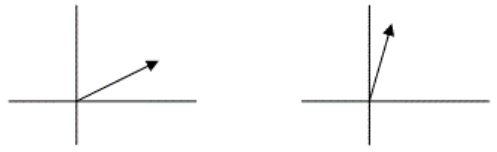(B)二维平面图像旋转示意图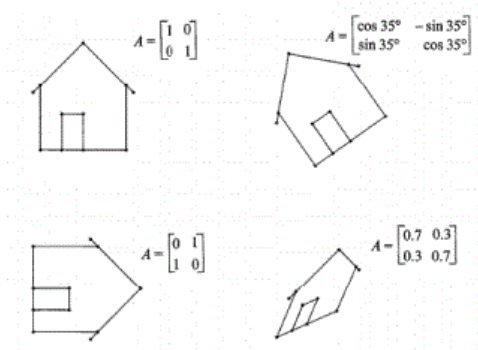这里其实是通过$$T(\mathbf{v})=A \mathbf{v}$$左乘一个矩阵$$A$$进行线性变换，对于旋转操作，这个矩阵为$$\left[\begin{array}{cc} \cos \theta & -\sin \theta \\ \sin \theta & \cos \theta \end{array}\right]$$。如果想让这个房子上下颠倒(沿着$$x$$轴翻转或者说镜像)，那就不是旋转操作，不过也还是线性操作，对应的矩阵为$$\left[\begin{array}{cc} 1 & 0 \\ 0 & -1 \end{array}\right]$$。

(3)$$T(\mathbf{v})=A \mathbf{v}$$

(1) 平面平移(Shift whole plane)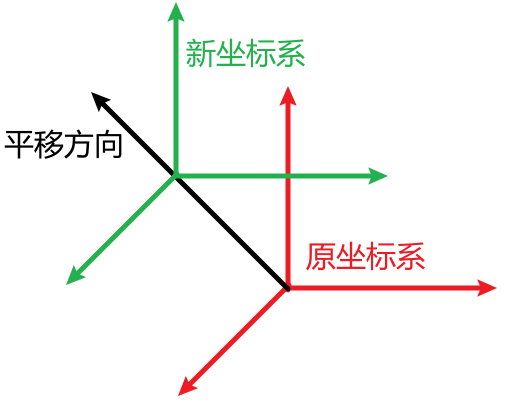(2) 求长度$$T(\mathbf{v})=\|\mathbf{v}\|$$

$$A\left[\begin{array}{c} 1 \\ 0 \\ \vdots \\ 0 \end{array}\right]=\left[\begin{array}{c} a_{11} \\ a_{21} \\ \vdots \\ a_{m 1} \end{array}\right]$$所得结果就是输出空间的坐标，即输出空间的基进行线性组合所需的系数。

## 仿射变换

xxx补充(1) 如何通俗地讲解「仿射变换」这个概念?—知乎
(2) 仿射变换及其变换矩阵的理解
(3) 计算机图形学—中科大

xx

## 向量空间-群-映射

xx

### 不变子空间

(1) $$\{0\}$$，因为每个线性映射都必有映射$$0 \mapsto 0$$；
(2) $$V$$，显然$$\mathbf{v} \in V \Longrightarrow T(\mathbf{v}) \in V$$；

(3) 令 $$\mathbf{v}$$ 为 $$T$$ 的一个特征向量，也即 $$T \mathbf{v}=\lambda \mathbf{v}$$ 。则 $$W=\operatorname{span}\{\mathbf{v}\}$$ 是 $$T$$ 不变的。

### 线性空间的直和分解

• $$W=\sum V_{i}$$是直和；
• 零向量的表法唯一；
• $$V_{i} \cap \displaystyle\sum_{j \neq i} V_{j}=\{0\}(i=1,2, \cdots, s)$$
• $$\text { dim }(W)=\sum \text { dim }\left(V_{i}\right)$$

• 子空间的和对应集合中的并集；
• 子空间的直和对应集合中的不交并；
• 子空间的$$0$$(零向量)对应集合中的空集；
• 向量空间中直和与和的区别 正如集合中并集和不交并的区别。

• 这其实是算子对角化的，推广到无限维空间也是成立的，比如拉普拉斯算子也可以进行这样的分解；
• 其实上面的直和分解的不变子空间就是各个特征向量所在的子空间，它们也是不变子空间；
• 矩阵的特征值要想说清楚还要从线性变换入手，把一个矩阵当作一个线性变换在某一组基下的矩阵，最简单的线性变换就是数乘变换，求特征值的目的就是看看一个线性变换对一些非零向量的作用是否能够相当于一个数乘变换，特征值就是这个数乘变换的变换比，这样的一些非零向量就是特征向量，其实我们更关心的是特征向量，希望能把原先的线性空间分解成一些和特征向量相关的子空间的直和，这样我们的研究就可以分别限定在这些子空间上来进行，这和物理中在研究运动的时候将运动分解成水平方向和垂直方向的做法是一个道理！
• 本质就是约化，把高维的问题约化到低维，把复杂的变换约化成简单的变换，降维过程往往和特征值/特征向量有关；
• 比方说随便写一个乱七八糟的方阵，你不知道怎么处理，事实上数学家a priori也不知道怎么处理(a priori不知道怎么翻译比较好，“先验”？)但是给你一个对角阵，你的思路估计会清晰很多，你知道对角阵代表的就是在不同的特征方向上做不同的伸缩变换(当然伸缩的系数可能是复数，这种情形对应到实平面里面的旋转＋伸缩)的一个复合。然后有一个奇迹出现了：很多矩阵，在你换一个角度(换一套坐标系)去观察以后，它其实就是个对角阵。你去把一个矩阵对角化的过程，就是去找那些特征方向，也就是一维的不变子空间的过程，也就是你去寻找最佳的“观察角度”的过程。

当然你不可能总是这么幸运。有些矩阵是不可对角化的，本质原因是他们有2维以上的“不可约”不变子空间(这里的不可约和表示论里面的不可约是一个意思)。在这种情形下，如果是复矩阵，你就应该去考虑所谓的Jordan标准型。非平凡的Jordan块当然比对角阵麻烦一点，但是比起原来那个乱七八糟的矩阵总是方便太多。在这种情形下你还是要去找所有的“不可约”不变子空间(Jordan标准型里的根子空间)，然后考虑这个矩阵在每个不变子空间上长什么样(Jordan块)。这个过程就好像你面对一个复杂的机器(让你不知所措的一个矩阵)，你先观察它的内在结构(寻找它的特征空间／不变子空间)，然后拿锤头起子或者别的什么工具(特征值、(广义)特征向量等知识)把它拆卸成一个个你可以理解的零件(把大矩阵分解成你可以理解的小矩阵块，比如Jordan块)，然后你就对原来那个机器的运作机理有所了解了。本质上是一种“分析”的思维方式(这里的分析不是数学分析意义上的分析，而是跟“综合”相对的那个分析)。—Yuhang Liu

• 集合里的元素，可能是数字，也可能是数组等；
• 运算，比如加法，乘法运算。

### 同构(Isomorphism)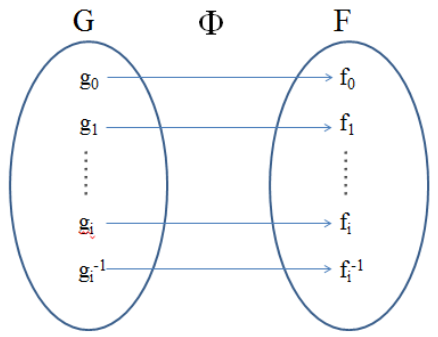• 从数学角度，两个同构的群有完全相同的结构，没有本质的区别；
• 空间反演群{E、I}与二阶循环群{ea}完全同构。
• 三阶置换群与 D群完全同构。
• 数二维空间的旋转群SO(2)和一维复数空间的旋转群U(1)同构的。

xxxxxx

### 同态(Homomorphism)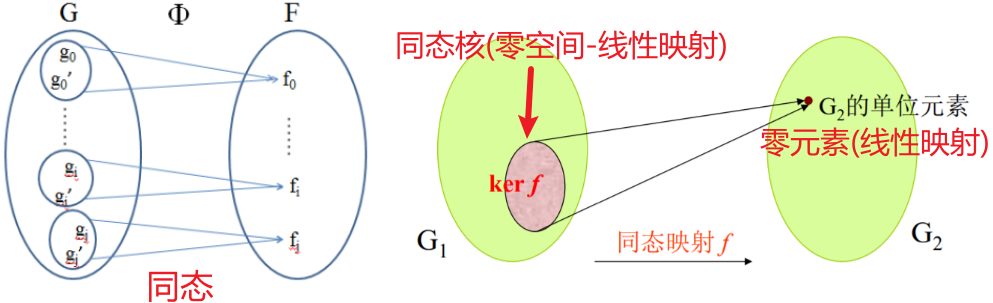• Homomorphisms of vector spaces are also called linear maps(线性映射)。
• 群同态在向量空间上的体现是线性映射。
• 群同态的核kernel体现为线性映射(矩阵)的零空间Null Space。

### 映射

• 函数$$f: \mathbb{R} \rightarrow \mathbb{R}: x \mapsto c x$$，the graph is a line through the origin.
• $$T(\boldsymbol{v})=\boldsymbol{v}+\boldsymbol{u}_{0}$$不是线性变换，因为$$T(\mathbf{0})\neq\mathbf{0}$$。
• The zero map $$\mathbf{x} \mapsto \mathbf{0}$$ between two vector spaces (over the same field) is linear.
• For real numbers, the map $$x \mapsto x^{2}$$ is not linear.
• Differentiation defines a linear map from the space of all differentiable functions to the space of all functions. It also defines a linear operator on the space of all smooth functions$$\frac{d}{d x}\left(c_{1} f_{1}(x)+c_{2} f_{2}(x)+\cdots+c_{n} f_{n}(x)\right)=c_{1} \frac{d f_{1}(x)}{d x}+c_{2} \frac{d f_{2}(x)}{d x}+\cdots+c_{n} \frac{d f_{n}(x)}{d x}$$
• A definite integral over some interval $$I$$ is a linear map from the space of all real-valued integrable functions on $$I$$ to $$\mathbb{R}$$. For example,$$\int_{a}^{b}\left[c_{1} f_{1}(x)+c_{2} f_{2}(x)+\cdots+c_{n} f_{n}(x)\right] d x=c_{1} \int_{a}^{b} f_{1}(x) d x+c_{2} \int_{a}^{b} f_{2}(x) d x+\cdots+c_{n} \int_{a}^{b} f_{n}(x) d x$$

• 函数 $$f: \mathbb{R} \rightarrow \mathbb{R}$$ ，其形式为 $$f(x)=2 x+1$$ ，是双射的。
• 指数函数 $$g: \mathbb{R} \rightarrow \mathbb{R}$$ ，其形式为 $$g(x)=e^{x}$$ ，不是双射。因为不存在一 $$\mathbb{R}$$ 内的 $$x$$ 使得 $$g(x)=-1$$ ，故 $$g$$ 非为双射。但若其陪域(Codomain，也叫到达域)改成正实数 $$\mathbb{R}^{+}=(0,+\infty)$$ ，则 $$g$$ 便是双射，其反函数为自然对数函数 $$\ln$$。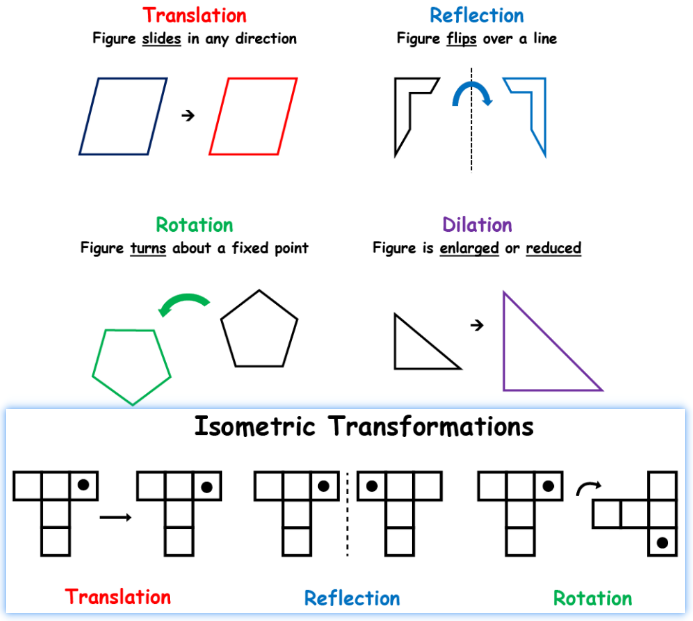• Translation (figure slides in any direction)
• Reflection (figure flips over a line)
• Rotation (figure turns about a fixed point)
• Dilation (figure is enlarged or reduced)

(1) 同构的向量空间—线代启示录
(2) 保長、保角與共形映射—线代启示录
(2) 如何理解同构的向量空间?—知乎
(3) 浅谈线性代数与群论的一致性-A  -B